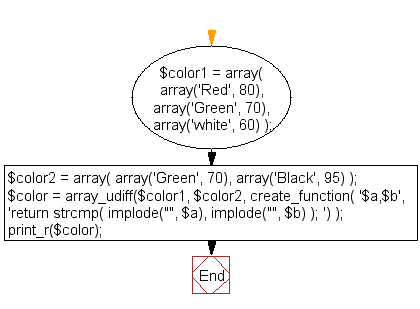﻿ PHP Array Exercise: Returns values of the first array that are not in the second array - w3resource# PHP Array Exercises : Returns values of the first array that are not in the second array

## PHP Array: Exercise-45 with Solution

Write a PHP script to do a multi-dimensional difference, i.e. returns values of the first array that are not in the second array.

Sample Solution:

PHP Code:

``````<?php
\$color1 = array( array('Red', 80), array('Green', 70), array('white', 60) );

\$color2 = array( array('Green', 70), array('Black', 95) );

\$color = array_udiff(\$color1, \$color2, create_function(
'\$a,\$b', 'return strcmp( implode("", \$a), implode("", \$b) ); ')
);

print_r(\$color);
?>
```
```

Sample Output:

```Array
(
 => Array
(
 => Red
 => 80
)

 => Array
(
 => white
 => 60
)

)
```

Flowchart:PHP Code Editor:

What is the difficulty level of this exercise?

﻿

## PHP: Tips of the Day

PHP: Extract numbers from a string

```\$str = 'In My Cart : 11 12 items';
preg_match_all('!\d+!', \$str, \$matches);
print_r(\$matches);
```